Open in App
Not now

# Polymorphism in R Programming

• Difficulty Level : Medium
• Last Updated : 06 Jan, 2023

R language is evolving and it implements parametric polymorphism, which means that methods in R refer to functions, not classes. Parametric polymorphism primarily lets you define a generic method or function for types of objects you haven’t yet defined and may never do. This means that one can use the same name for several functions with different sets of arguments and from various classes. R’s method call mechanism is generics which allows registering certain names to be treated as methods in R, and they act as dispatchers.

## Generic Functions

Polymorphism in R can be obtained by the generics. It allows certain names to be treated as methods and they act as dispatchers. Let’s understand with the help of plot() function and summary function. In R programming the plot() and summary() functions return different results depending on the objects being passed to them and that’s why they are generic functions that implement polymorphism.

## plot() in R

plot() is one of the generic functions that implement polymorphism in R. It produces a different graph if it is given a vector, a factor, a data frame, etc. But have you ever wondered how does the class of vectors or factors determine the method used for plotting?  Let’s see the code for the plot function.

Example: Code of plot function

## R

 `plot`

Output:

```function (x, y, ...)
UseMethod("plot")```

We can see that the body of the plot function contains only one expression and that is UseMethod(“plot”). Let’s see the definition of UseMethod with the help of help() function.

Example: Definition of the help() method.

## R

 `help``(UseMethod)`

Output: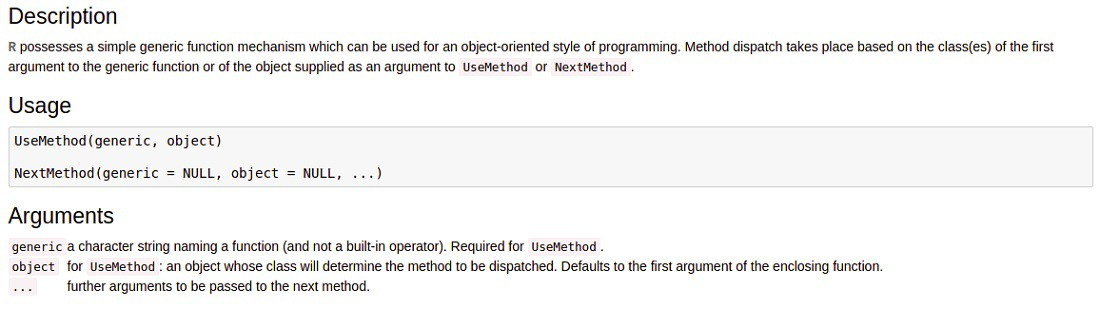From the above output, we can see that UseMethod takes two parameters generic and object.

• The generic is the string name which is the name of the function (plot in this case).
• This is an object whose class will determine the method that will be “dispatched,” It means the object for which the generic method will be called.

The UseMethod then searches for the suitable plot function that is needed to be called by creating a string of the type plot.object. We can also see all the available methods for the plot function.

Example:

## R

 `methods``(plot)`

Output: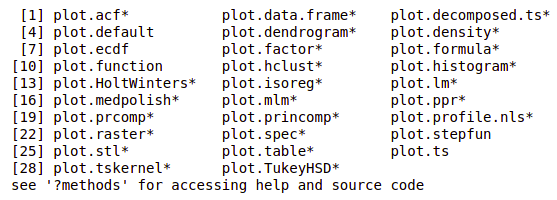Let’s see how plot() function taking arguments and displaying different outputs

### Input is one numeric vector

In this example let’s take a single numeric vector inside plot() function as a parameter.

## R

 `# R program to illustrate` `# polymorphosim`   `# X Window System Graphics (X11)` `X11``(width = 15, height = 13)`   `# The runif() function generates` `# random deviates of the uniform distribution` `x <- 3 * ``runif``(40) + (1:30)` `par``(mar = ``c``(20, 20, 1, 1))`   `# type='l' is used  to connect the points ` `# of the scatter plots with lines.` `plot``(x, type = ``'l'``, col = ``'#343deb'``)`   `# We can do  mouse click or enter pressed` `z <- ``locator``(1)`

Output: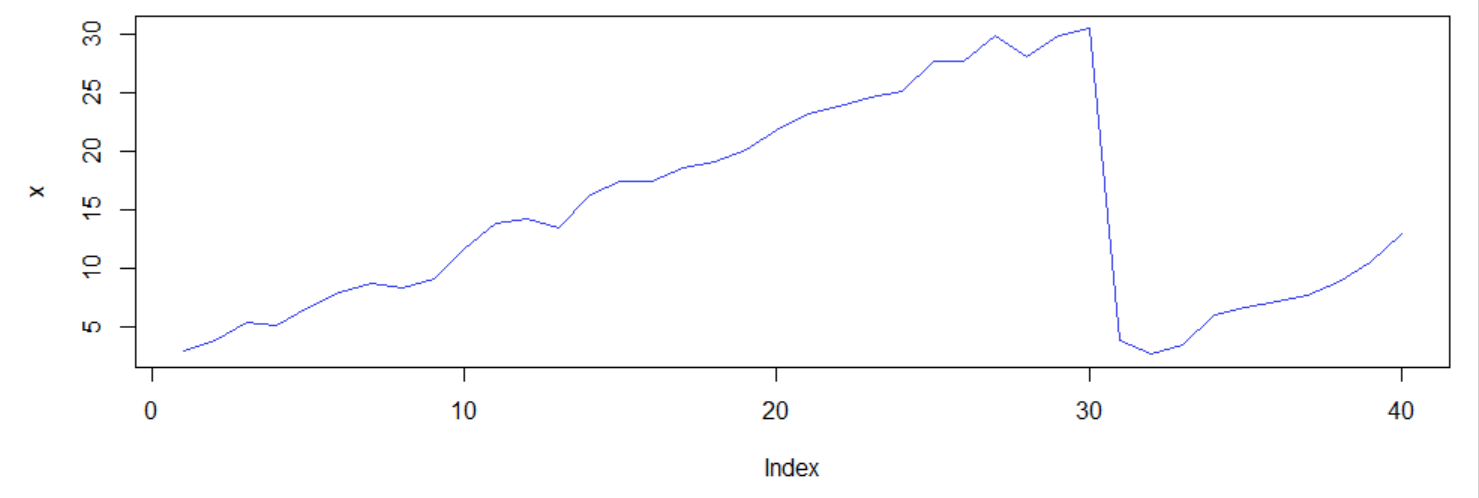### Inputs are two numeric vectors

We need to pass two vector parameters and it produces a scatter plot accordingly.

## R

 `# R program to illustrate` `# polymorphosim`   `# X Window System Graphics (X11)` `X11``(width = 5, height = 3)`   `# The runif() function generates random` `# deviates of the uniform distribution` `x <- ``runif``(20)` `y <- ``runif``(20) * x` `par``(mar = ``c``(2, 2, 0.3, 0.3))`   `# type = 'p' means as points, the output comes as scattered` `# pch stands for plot character. pch = 16 we get . character` `plot``(x, y, type = ``'p'``, pch = 16, col = ``'#ab1ab0'``)`   `#Either mouse press or enter key press wait` `z <- ``locator``(1)`

Output: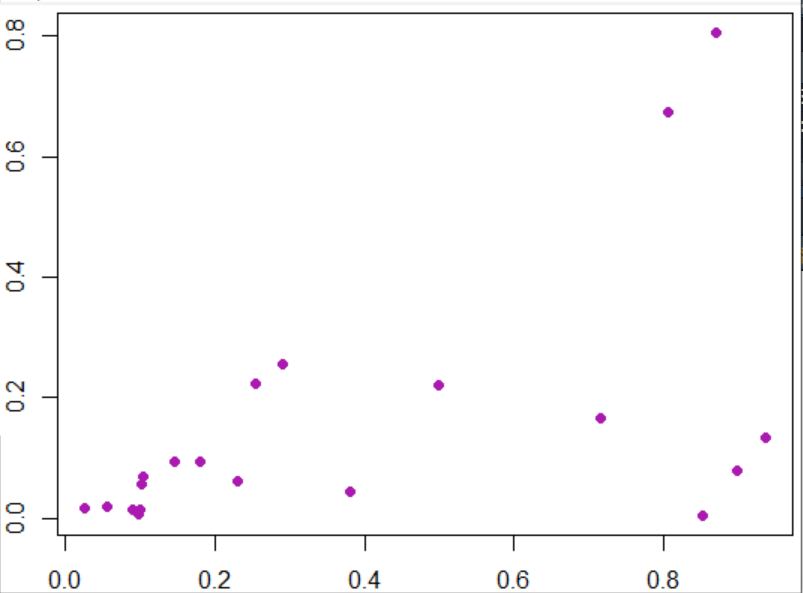### Input is a factor

If we passed factor as arguments then we get a bar chart pattern.

## R

 `# R program to illustrate` `# polymorphosim`   `# X Window System Graphics (X11)` `X11``(width = 5, height = 8)`   `# here fruits names are passed and barchart is produced as output` `f <- ``factor``(``c``(``'apple'``, ``'orange'``, ``'apple'``, ``'pear'``, ``'orange'``,` `              ``'apple'``, ``'apple'``, ``'orange'``))` `par``(mar = ``c``(2, 2, 0.6, 0.6))`   `# Using plot()` `plot``(f, col = ``'#8f4c91'``)` `z <- ``locator``(1)`

Output: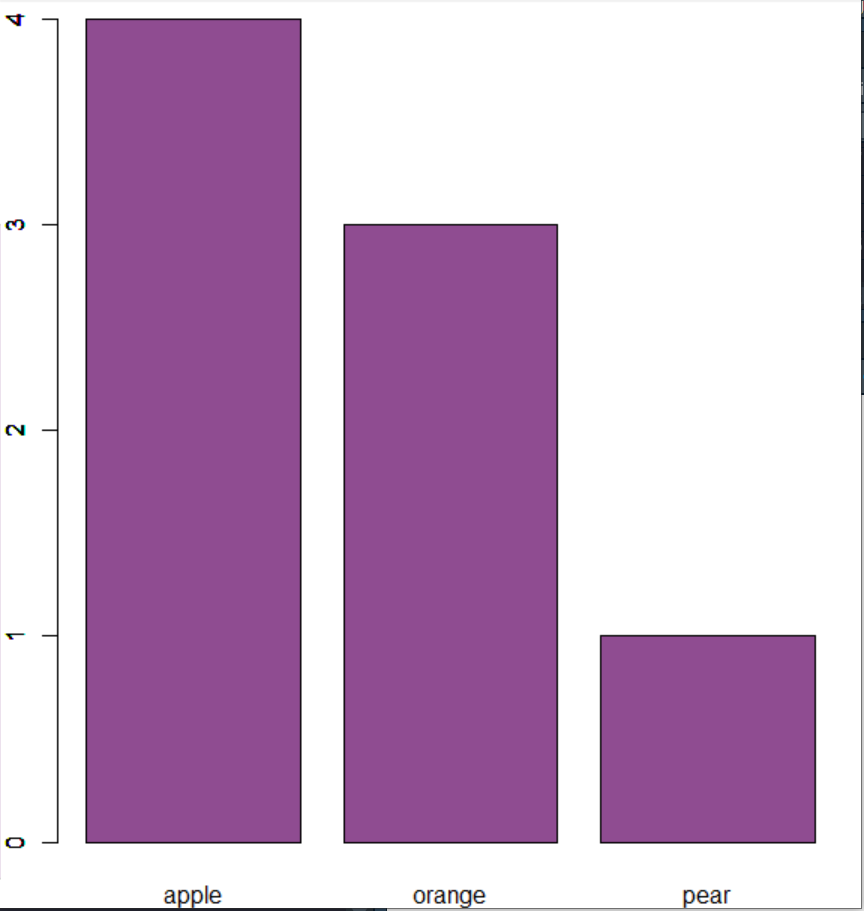### Input is a data frame

The Plot function takes the data frame as an argument and each variable of the data frame is plotted against each other.

## R

 `# R program to illustrate` `# polymorphosim`   `# X Window System Graphics (X11)` `X11``(width = 6, height = 6)`   `set.seed``(280870)` `x <- ``c``(4, 3, 1, 2, 2, 4, 6, 4, 5, 5, ` `       ``4, 4, 5, 4, 4, 8, 4, 1, 2, 7)` `y <- x * ``rnorm``(20, 1, 0.3)` `z <- x * y`   `# Taking a data frame` `df <- ``data.frame``(x, y, z)` `par``(mar = ``c``(0.1, 0.1, 0.1, 0.1))`   `# Using plot()` `plot``(df, col = ``'#a832a6'``, pch = 16, cex = 1.5)` `z <- ``locator``(1)`

Output: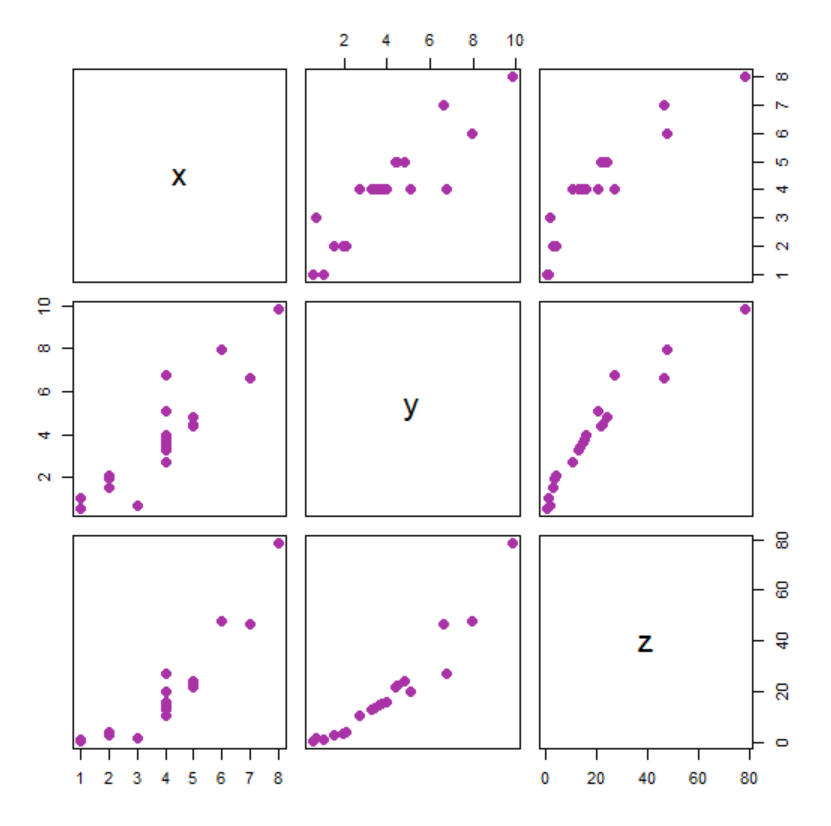## summary() in R

It is also a generic function that implements polymorphism in R. It is used to produce result summaries of the results of various model fitting functions.

Example 1:

## R

 `# R program to illustrate` `# polymorphosim`   `# Rainbow colors and let us see summary of it` `colors <- ``c``(``"violet"``, ``"indigo"``, ``"blue"``, ``"green"``,` `            ``"yellow"``, ``"orange"``, ``"red"``)` `summary``(colors)`

Output:

```Length     Class      Mode
7      character character ```

Example 2:

Let us check for summarized results for state.region. In R it usually displays what are the regions available under “Northeast”, “South”, “North Central”, and “West”. Using summary() function either one can pass state.region as 1st parameter and as a second step, (optionally) pass “maxsum” argument. “maxsum” indicates how many levels should be shown for factors in output.

## R

 `# R program to illustrate` `# polymorphosim`   `state.region`   `# Provides summarised results under each region` `summary``(state.region) `   `# As maxsum is given as 3, totally we should have 3 regions` `# But here we have 4 regions and hence highest count region,` `# next highest count region is displayed and the other ` `# regions are clubbed under Other` `summary``(state.region, maxsum = 3)`

Output:

```> state.region
 South         West          West          South         West          West
 Northeast     South         South         South         West          West
 North Central North Central North Central North Central South         South
 Northeast     South         Northeast     North Central North Central South
 North Central West          North Central West          Northeast     Northeast
 West          Northeast     South         North Central North Central South
 West          Northeast     Northeast     South         North Central South
 South         West          Northeast     South         West          South
 North Central West
Levels: Northeast South North Central West

> summary(state.region)
Northeast         South North Central          West
9            16            12            13

> summary(state.region, maxsum = 3)
South    West (Other)
16      13      21 ```

Example 3:

If the data set is very large then let’s have a look at how the summary() function works.

## R

 `# R program to illustrate` `# polymorphosim`   `# 10 different data sets are taken using stats::rnorm` `x <- stats::``rnorm``(10)` `x`   `# Let us cut the dataset to lie between -3 and 3 and ` `# in this case, it will be` `# (-3,-2] (-2,-1] (-1,0] (0,1] (1,2] (2,3]` `c <- ``cut``(x, breaks = -3:3)` `c`   `# Summarized the available dataset under the given levels` `summary``(c)`

Output:

```> x
  0.66647846 -0.29140286 -0.29596477 -0.23432541 -0.02144178  1.56640107  0.64575227
 -0.23759734  0.73304657 -0.04201218

> c
 (0,1]  (-1,0] (-1,0] (-1,0] (-1,0] (1,2]  (0,1]  (-1,0] (0,1]  (-1,0]
Levels: (-3,-2] (-2,-1] (-1,0] (0,1] (1,2] (2,3]

> summary(c)
(-3,-2] (-2,-1]  (-1,0]   (0,1]   (1,2]   (2,3]
0       0       6       3       1       0 ```

Till now, we have described the plot() and summary() function which is a polymorphic function. By means of different inputs, plot() function behavior is changing and producing outputs. Here we can see the polymorphism concept. Similarly, in summary(), function, by means of varying parameters, the same method is applicable to provide different statistical outputs. Now let’s create our own generic methods.

## Creating Generic Method

Let’s create a class bank and let’s try to create our own display() method that will use the print() method and will display the content of the class in the format specified by us.

For doing this we first have to create a generic display() function that will use the UseMethod function.

## R

 `display <- ``function``(obj){` `    ``UseMethod``(``"print"``)` `}`

After creating the generic display function let’s create the display function for our class bank.

## R

 `print.bank<-``function``(obj)` `{` `    ``cat``(``"Name is "``, obj\$name, ``"\n"``)` `    ``cat``(obj\$account_no, ``" is the Acc no of the holder\n "``)` `    ``cat``(obj\$saving, ``" is the amount of saving in the account \n "``)` `    ``cat``(obj\$withdrawn, ``" is the withdrawn amount\n"``)` `}`

Now, let’s see the output given by this function

## R

 `x <- ``list``(name =``"Arjun"``, account_no = 1234,` `        ``saving = 1500, withdrawn = 234)` `class``(x)<-``"bank"`   `display <- ``function``(obj){` `    ``UseMethod``(``"print"``)` `}`   `print.bank<-``function``(obj)` `{` `    ``cat``(``"Name is "``, obj\$name, ``"\n"``)` `    ``cat``(obj\$account_no, ``" is the Acc no of the holder\n "``)` `    ``cat``(obj\$saving, ``" is the amount of saving in the account \n "``)` `    ``cat``(obj\$withdrawn, ``" is the withdrawn amount\n"``)` `}`   `display``(x)`

Output:

```Name is  Arjun
1234  is the Acc no of the holder
1500  is the amount of saving in the account
234  is the withdrawn amount```

My Personal Notes arrow_drop_up
Related Articles